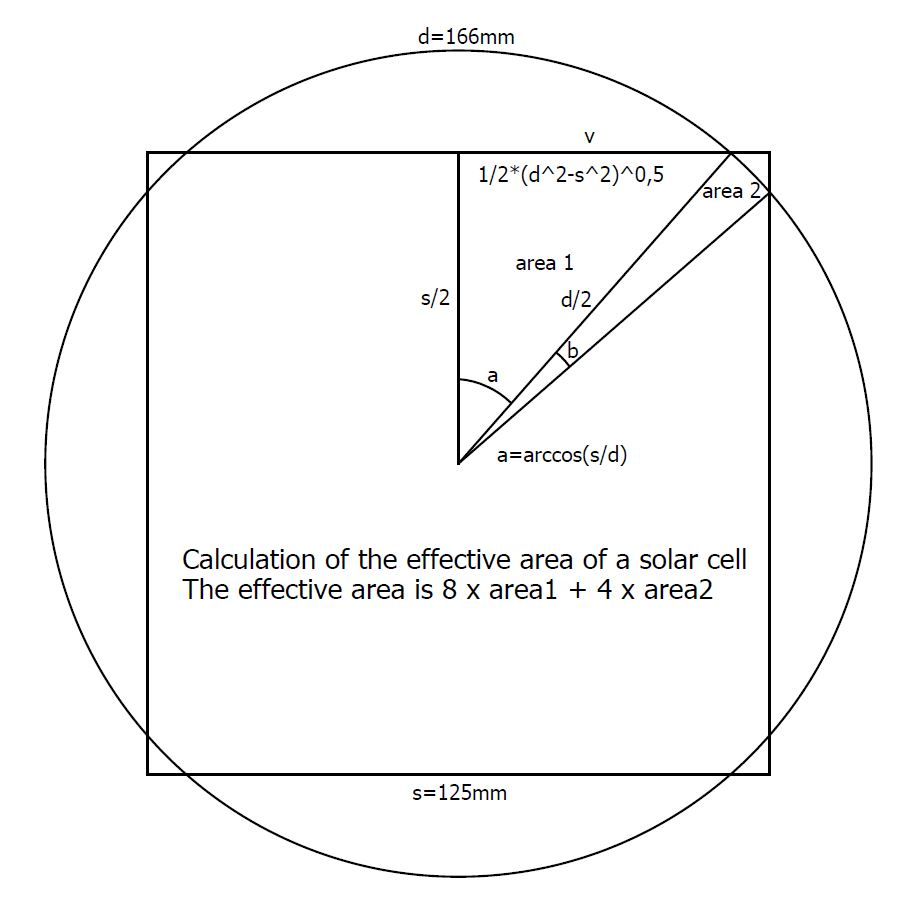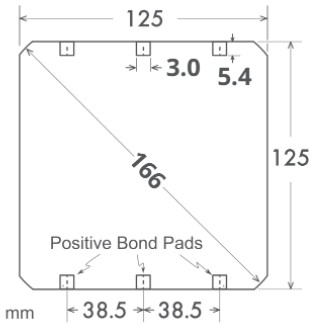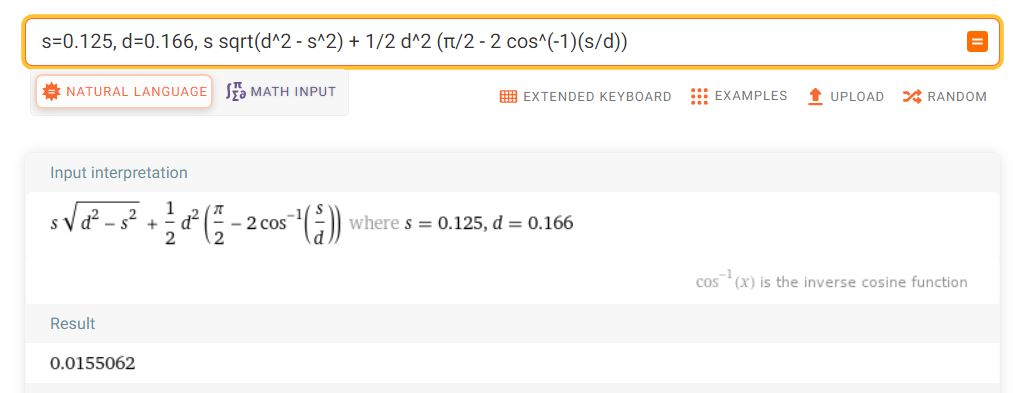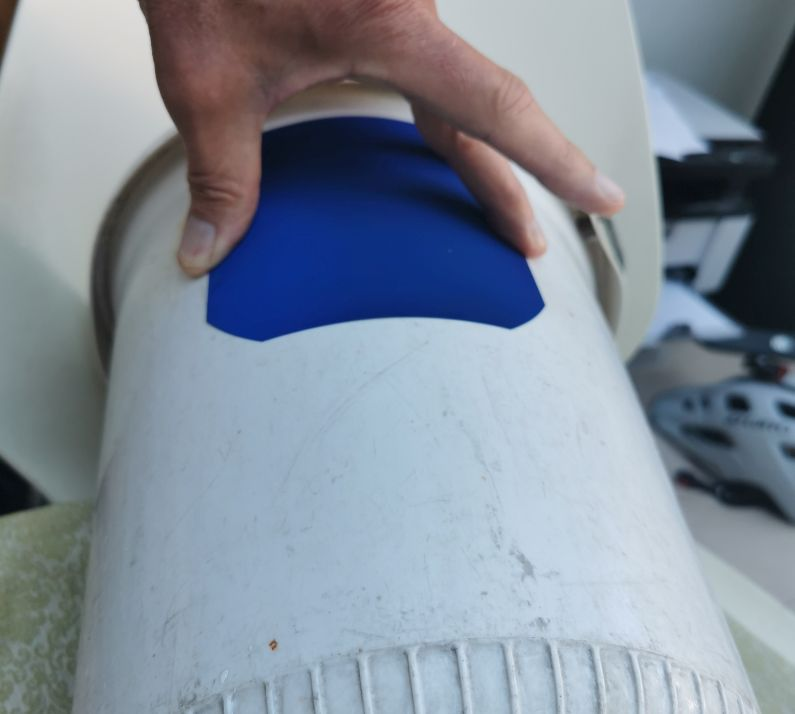Oproep: Ik zoek iemand die kan zorgen voor de continuïteit van deze website

# Using Sunpower solar cells at the World Solar Challenge

Published: 30 May 2022
Last updated: 05 January 2023

In this article I tell something about the use of solar cells at the World Solar Challenge and other contests.

### How to calculate the effective area of a Sunpower solar cell

For the solar panels, there is a maximum allowed solar panel area of, for example, 4 m2, and for this you have to know the exact area of your solar cells. It concerns the active surface of the solar cells (so it makes no sense to make the cells square if you know what I mean). I couldn't find any calculation anywhere on the internet and therefore I have calculated it for everyone and once and for all.

To calculate, I divided the PV cell into triangles (area 1) and pie segments (area 2). The effective area is 8 * area1 + 4 * area2. I've used Wolfram Alpha which can simplify the formula automatically.Calculation effective area Sunpower solar cell
The initial long formula was
4((PI /2-2(arccos(s/d)))/(2PI)d^2(PI/4))+8(s/2)(1/2(d^2-s^2)^0.5)/2
s=wide, d=diameter
The simplified formula is
s sqrt(d^2 - s^2) + 1/2 d^2 (π/2 - 2 cos^(-1)(s/d))

### Example for Maxeon gen 3 solar cellsUsing Sunpower solar cells at the World Solar Challenge
s=0.125, d=0.166

If you know the size and the diameter of the solar cell, you can use wolframalpha to calculate the effective area, just use this link
https://www.wolframalpha.com/input?i=s%3D0.125%2C+d%3D0.166%2C++s+sqrt%28d%5E2+-+s%5E2%29+%2B+1%2F2+d%5E2+%28%CF%80%2F2+-+2+cos%5E%28-1%29%28s%2Fd%29%29Calculation effective area solar cell with Wolfram Alpha

The area is 0.0155062 m2

### Here is the formula for Excel

s*(d^2-s^2)^(0,5)+1/2*d^2*(PI()/2-2*BOOGCOS(s/d))

### This text is from the World Solar Challenge 2023

Example calculation: The area of a SunPower cell with a width of 125 mm and a diagonal diameter of 166 mm is less than 0.0155063 m2, and so the area of 257 cells is less than 3.9852 m2 and the area of 322 cells is less than 4.9931 m2.

### Solar cell minimum bend radius

I have measured the minimum bend radius of the 125mm solar cell while it just doesn't break, this is about 110mm.Maxeon Sunpower solar cell bend radius 110mm